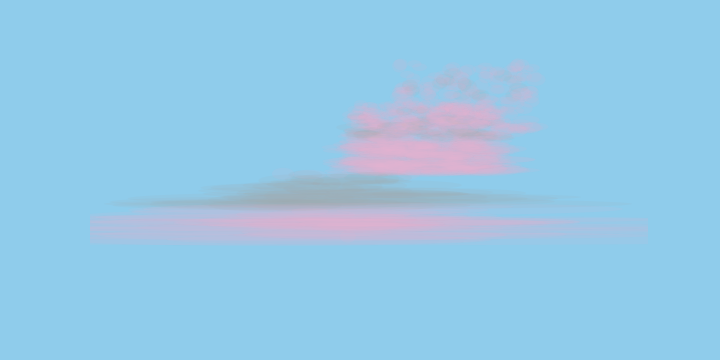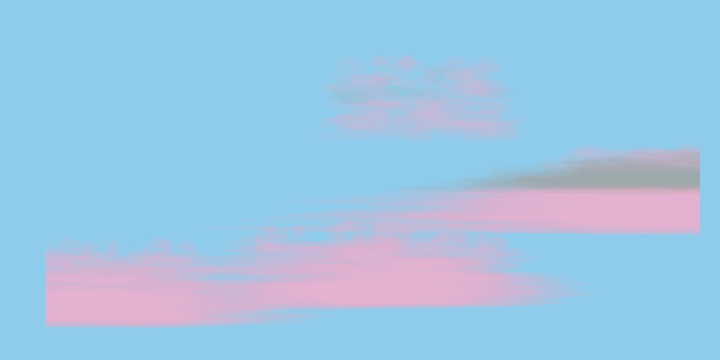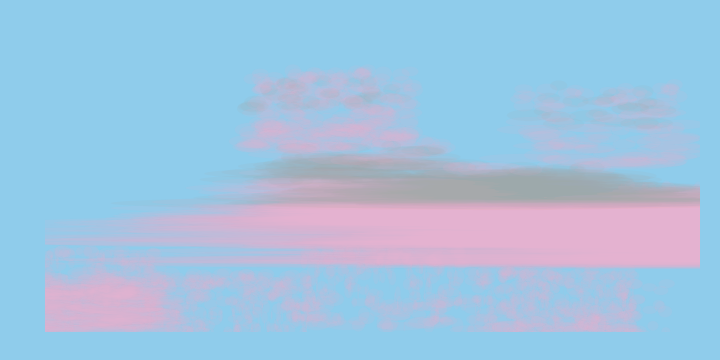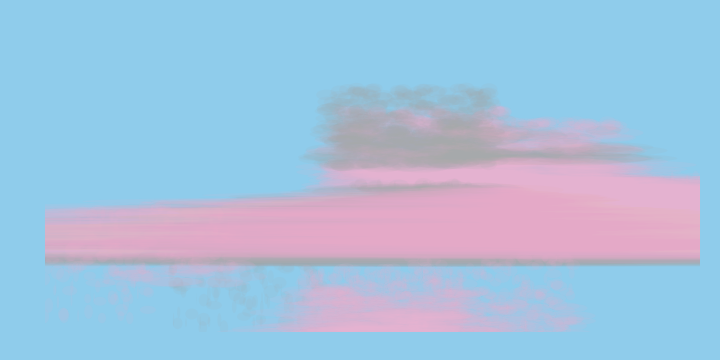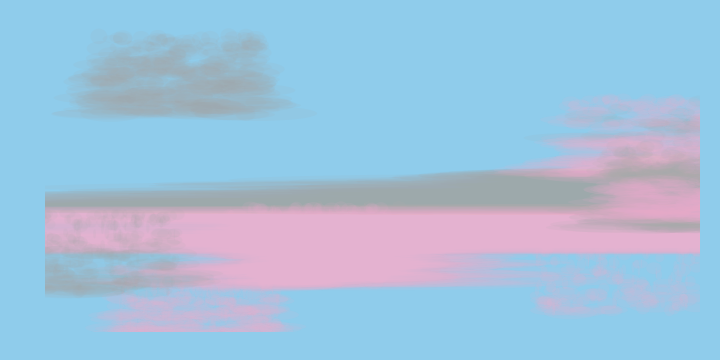# Procedural art: Clouds

(18 Jul 2019) python art

My first original piece of generative art inspired by a pretty sunset last night. The texture of the clouds is achieved by overlaying transparent ellipses.

``````    import numpy as np
import matplotlib.pyplot as plt
from matplotlib.patches import Ellipse
``````

Overlay small ellipses, control the color depending on the height.

``````    def draw_cloud(x,y,ax,numiter=50):
colorful = True
r = np.random.random()
if r> 0.5:
colorful = False
# Center the cloud at x, y
xra = [x-50,x+50]
yra = [y-25, y+25]
# Sample width depending on how "dense" you
# want the cloud to appear. More dense, wider
# less dense, narrower clouds.
wi = [10, np.random.uniform(15,5*(numiter/10))]
hi = [5, np.random.uniform(7,10)]
colors = ['#edb2d0', # pink
'#a6a9ab'  # gray
]
for i in range(numiter):
# sample the center of the patch
yc = np.random.uniform(yra, yra)
xc = np.random.uniform(xra, xra)
h = np.random.uniform(hi, hi)
# Make the width narrower as you go up the cloud.
w = np.random.uniform(wi,wi+ wi*(y+25-yc)/(y+25))
if colorful:
c = colors
# Clouds become gray towards the top with some prob.
if yc > y:
coin = np.random.random()
if coin > (yc)/(y+25):
c = colors
else:
# draw a gray cloud
c = colors

# Draw the ellipse
e = Ellipse(xy=(xc,yc),
width=w,
height=h,
alpha=0.1,
edgecolor=None,
facecolor=c)
``````

Display

``````    plt.close()
sky = '#8fcceb'#'#a2d9f5' #'#5fafed' #
f, ax = plt.subplots(1,1,figsize=(10,5), facecolor=sky)
####################
# Parameters
num_clouds = 10
canvasx = 200
canvasy = 100
####################
for i in range(num_clouds):
draw_cloud(int(np.random.uniform(-canvasx, canvasx)),
int(np.random.uniform(-canvasy, canvasy)),
ax,
numiter=int(np.random.uniform(100,500)))
ax.set_xlim([-canvasx,canvasx])
ax.set_ylim([-canvasy,canvasy])
ax.axis('off')
plt.tight_layout()
plt.savefig('clouds.png', facecolor=f.get_facecolor(), transparent=True)
``````